# Angular Momentum Assignment Help

## Angular Momentum

Consider a particle P of mass m, having position vector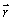, rotating about the z-axis. If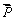is the momentum of the particle, then its angular momentum l is defined as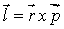The magnitude of angular momentum is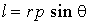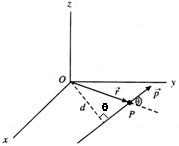and its direction is normal to the plane containingand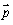, i.e., along the z-axis in this case.

Simplifying l = P (r sinq) = Pd, where d is perpendicular distance of the line of direction of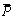. From (O).

If we have a collection of particles or a rigid body, the total angular momentum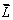will be the vector sum of the angular momentum of the individual particles.

## Angular Momentum Assignment Help By Online Tutoring and Guided Sessions at AssignmentHelp.Net

### Homework Help For Angular Momentum

assignmenthelp.net provides best Online Assignment Help service in Physics for all standards. Our Tutor provide their high quality and optimized Tutorial help to fulfill all kind of need of Students.